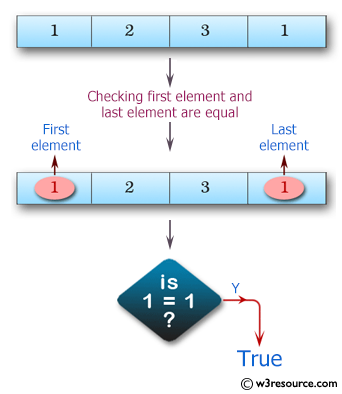﻿ Swift Array Programming Exercise: Check whether the first element and the last element of a given array of integers are equal - w3resource# Swift Array Programming Exercises: Check whether the first element and the last element of a given array of integers are equal

## Swift Array Programming: Exercise-2 with Solution

Write a Swift program to check whether the first element and the last element of a given array of integers are equal. The array length must be 1 or more.

Pictorial Presentation:Sample Solution:

Swift Code:

``````func check_first_last(_ arra: [Int]) -> Bool {
guard arra.count > 0 else
{
return false
}
if arra.first == arra.last
{
return true
} else
{
return false
}
}
print(check_first_last([1, 2, 3]))
print(check_first_last([1, 2, 3, 1]))
print(check_first_last([1, 2, 2, 1]))
print(check_first_last())
```
```

Sample Output:

```false
true
true
true
```

Swift Programming Code Editor:

Improve this sample solution and post your code through Disqus

What is the difficulty level of this exercise?

﻿